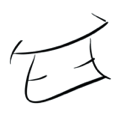# Ellipse — Html5 Canvas

There is no native method to draw an ellipse on a HTML5 canvas, and there are many different ways to accomplish it. In the past I’ve needed to draw a symmetrical ellipse, and thankfully there’s an easy way using scale transforms. After all an ellipse just a skewed\scaled circle.

To draw a circle using the HTML5 canvas API you would arc around a center point at a specific radius from 0° to 360° degrees. Written in code this looks as follows;

`var start = 0;var end = 2 * Math.PI; // 360 degrees is equal to 2π radianscontext.beginPath();context.arc(centerX, centerY, radius, start, end, clockwise);context.closePath();`

Note: The API uses radians and not degrees. So here we use 2π radians which is equal to 360° degrees.

To draw our ellipse, we need to calculate a scaling ratio for the height and width, and then apply this before calling the arc() method. We can do this by dividing the desired height and width by the longest of the two (height or width). Since the longest will be divided by itself, the result will be 1 and so the longest axis won’t be scaled. If the desired height and width are 75 and 170 respectively the circle will be scaled vertically by (75 / 150) = 0.5 = 50%. Let’s look at the code.

`var start = 0;var end = 2 * Math.PI; // 360 degrees is equal to 2π radiansvar circumference = Math.max(width, height);var scaleX = width / circumference;var scaleY = height / circumference;context.save();context.translate(centerX, centerY);context.scale(scaleX, scaleY);context.beginPath();context.arc(0, 0, radius, start, end, clockwise);context.closePath();context.restore();`

Since the scale will also be applied to the arc’s x and y coordinates, I've applied these with a transform prior to, applying the scale.

Note: The scale will also apply for the stroke which would give us thinner lines on some of the edges, so in this example I have restored the original context before calling the stroke() method.

--

--

--

## More from InfectiousGrin

http://infectiousgr.in

Love podcasts or audiobooks? Learn on the go with our new app.## InfectiousGrin

http://infectiousgr.in

## Designing an Automated Teller Machine (ATM) for Children